# Free precession of Earth

It is known that the Earth's axis of rotation is very slightly inclined to its symmetry axis (which passes through the two geographic poles). The angle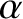is approximately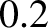seconds of an arc (which corresponds to a distance of about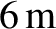on the Earth's surface). The ratio of the terrestrial moments of inertia is about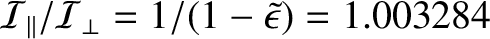, as determined from luni-solar precession. (See Appendix F.) Hence, from Equation (8.40), the precession rate of the angular velocity vector about the symmetry axis, as viewed in a geostationary reference frame, is(8.61)

giving a precession period of(8.62)

[Of course,(sidereal) day.] The observed period of precession is about 434 days (Yoder 1995). The disagreement between theory and observation is attributed to the fact that the Earth is not perfectly rigid (Bertotti et al. 2003). An improved treatment of the free precession of the Earth that takes into account its lack of complete rigidity is given in Appendix E.

The Earth's symmetry axis subtends an angle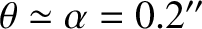[see Equation (8.44)] with its angular momentum vector, but it lies on the opposite side of this vector to the angular velocity vector. This implies that, as viewed from space, the Earth's angular velocity vector is almost parallel to its fixed angular momentum vector, whereas its symmetry axis subtends an angle of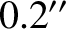with both vectors and precesses about them. The (theoretical) precession rate of the Earth's symmetry axis, as seen from space, is given by Equation (8.58):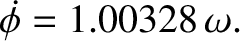(8.63)

The associated precession period is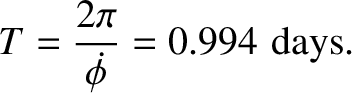(8.64)

The free precession of the Earth's symmetry axis in space, which is known as the Chandler wobble—because it was discovered by the American astronomer S.C. Chandler (1846–1913) in 1891—is superimposed on a much slower forced precession, with a period of about 26,000 years, caused by the small gravitational torque exerted on the Earth by the Sun and Moon, as a consequence of the Earth's slight oblateness. (See Section 8.10.)